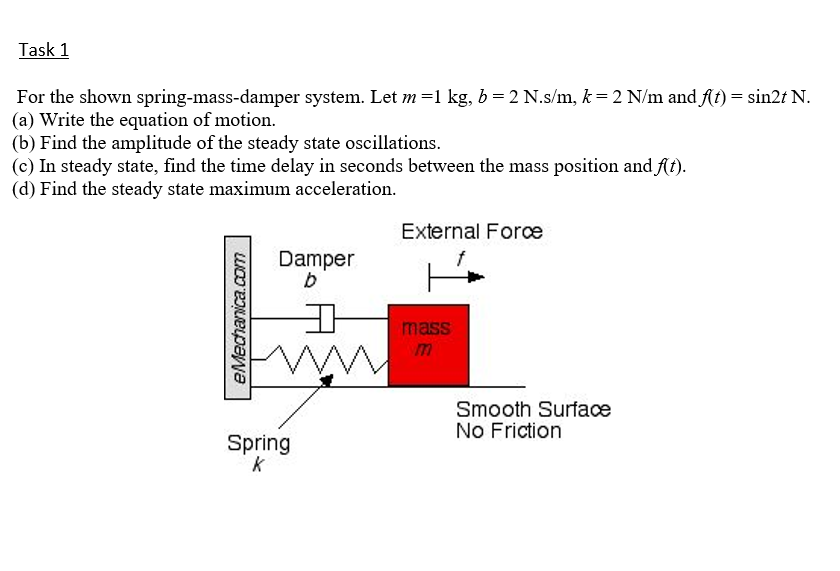# Task 1 For the shown spring-mass-damper system. Let m=1 kg, b = 2 N.s/m, k = 2 N/m and f(t) = sin2t N. (a) Write the equation of motion. (b) Find the amplitude of the steady state oscillations. (c) In steady state, find the time delay in seconds between the mass position and At). (d) Find the steady state maximum acceleration.

Questionhelp_outlineImage TranscriptioncloseTask 1 For the shown spring-mass-damper system. Let m=1 kg, b = 2 N.s/m, k = 2 N/m and f(t) = sin2t N. (a) Write the equation of motion. (b) Find the amplitude of the steady state oscillations. (c) In steady state, find the time delay in seconds between the mass position and At). (d) Find the steady state maximum acceleration. fullscreen

1 Rating

### Want to see this answer and more?

Experts are waiting 24/7 to provide step-by-step solutions in as fast as 30 minutes!*

*Response times vary by subject and question complexity. Median response time is 34 minutes and may be longer for new subjects.
Tagged in
EngineeringMechanical Engineering

### Mechanical Vibrations